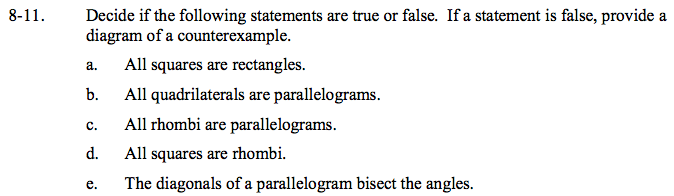Home > GC > Chapter 8 > Lesson 8.1.1 > Problem8-11

8-11.
1. Decide if the following statements are true or false. If a statement is false, provide a diagram of a counterexample. Homework Help ✎

1. All squares are rectangles.

2. All quadrilaterals are parallelograms.

3. All rhombi are parallelograms.

4. All squares are rhombi.

5. The diagonals of a parallelogram bisect the angles.Consider the definitions of the polygons, not just the typical examples.
If necessary, review definitions in the glossary.

True, a rectangle is a quadrilateral with four right angles and a square is a quadrilateral with four right angles and four congruent sides.

False, quadrilaterals can exist without parallel sides, like a kite or a trapezoid.
Remember a parallelogram has 2 sets of parallel sides.